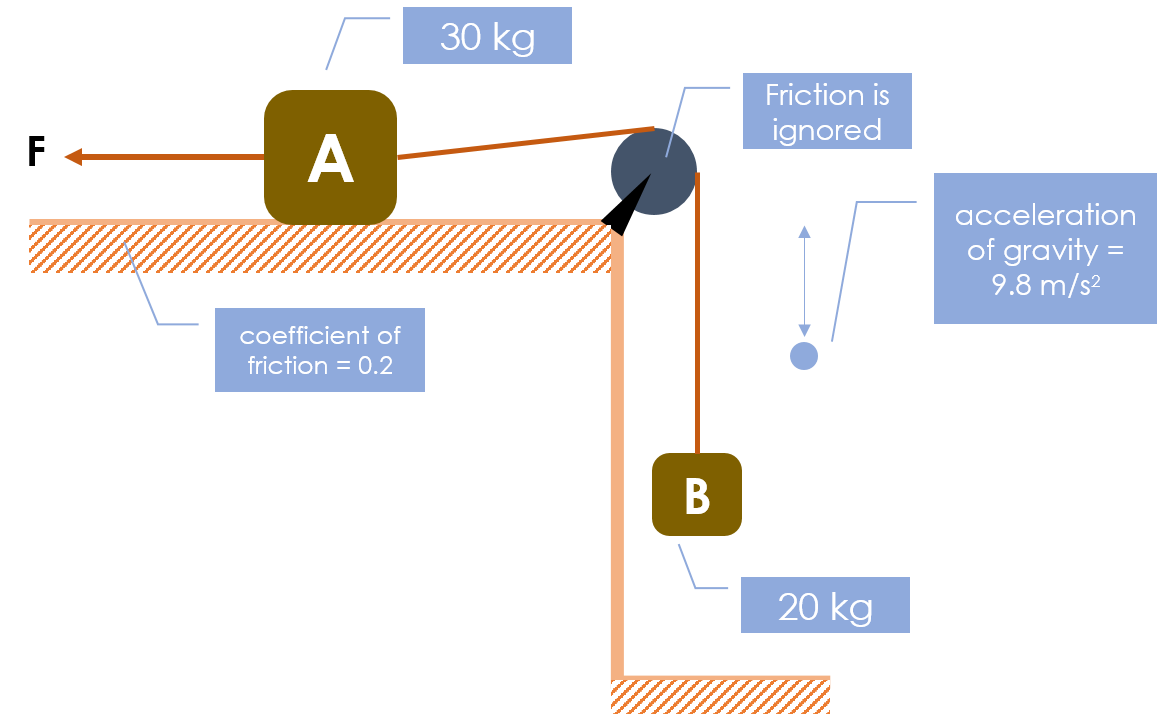# What is the force?How much force (N) are given to A, so system moved to the left with acceleration 3 m/s^2 ?

Please first obtain a general equation, then replaced one decimal

This problem is not original, the original problem has a bug The original problem is ---> Original Problem

×